Next: Experiments and results Up: Affine-to-Euclidean calibration Previous: General resolution

### Degenerate cases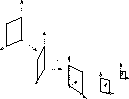Figure 1: example of critical motion : vertical rotation axis

Horizontal rotation axis. We have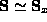and so,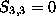and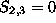(see eq:Sx) : eq:sys3 gives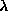and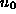and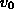can then be computed with eq:sys5 and eq:sys6.

if we impose r=0, eq:sys2 gives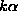. However, eq:sys1 can't give eitheror. if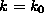is considered,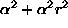and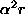can be calculated directly and solved to give r,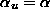and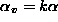.

Vertical rotation axis. This is the case when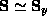, which is similar to the previous one. We have.

if r=0, we have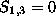and then all parameters except k andcan be evaluated. if,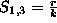leads to a total resolution of calibration

Rotation axis orthogonal to the image plane. We have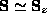(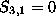and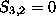).

It is the worst critical case in so far as,, k and r can be calculated, butremains always undetermined, whatever the constraint may be.

As a conclusion, we saw that the problem of affine-to-Euclidean calibration could be easily solved in particular cases (single motion, all parallel axes rotations).

We also showed that using the constraintallowed us to avoid critical cases : there remains then just one real critical motion (rotation axes orthogonal to the image plane).

Bob Fisher
Mon Dec 7 13:48:06 GMT 1998